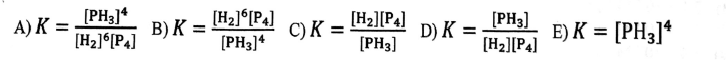# Problem: Choose the answer which gives the correct equilibrium constant expression for the reaction shown below. 6 H2(g) + P4(g) ⇌ 4 PH3(g)

###### FREE Expert Solution
84% (396 ratings)
###### Problem Details

Choose the answer which gives the correct equilibrium constant expression for the reaction shown below.

6 H2(g) + P4(g) ⇌ 4 PH3(g)What scientific concept do you need to know in order to solve this problem?

Our tutors have indicated that to solve this problem you will need to apply the Equilibrium Expressions concept. If you need more Equilibrium Expressions practice, you can also practice Equilibrium Expressions practice problems.

What is the difficulty of this problem?

Our tutors rated the difficulty ofChoose the answer which gives the correct equilibrium consta...as medium difficulty.

How long does this problem take to solve?

Our expert Chemistry tutor, Sabrina took 2 minutes and 9 seconds to solve this problem. You can follow their steps in the video explanation above.

What professor is this problem relevant for?

Based on our data, we think this problem is relevant for Professor Del Negro's class at NCSU.